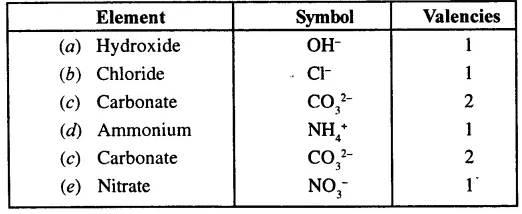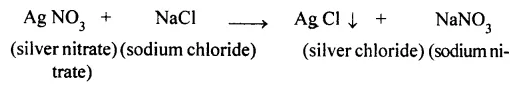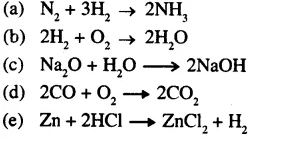Saturday, March 25, 2023

# Language of Chemistry ICSE Class-8th Concise Selina Chemistry Solutions

Language of Chemistry ICSE Class-8th Concise Selina Chemistry Solutions Chapter-5. We Provide Step by Step Answer of Objective, True False , Fill in the blanks , Multiple choice Short / Long Answer Type of Exercise-5 Language of Chemistry. Visit official Website CISCE for detail information about ICSE Board Class-8.

## Language of Chemistry ICSE Class-8th Concise Selina Chemistry Solutions

### Exercise

#### Question 1.

Define:
(b) Valency
(c) Molecular formula
(a) Radical: A radical is an atom of an element or a group of atoms of different elements that behaves as a single unit with a positive or negative charge on it.
(b) Valency: It is the number of electrons donated or accepted by the valence shell of an atom during chemical combination.
(c) Molecular formula: It is a symbolic representation of a molecule. It shows the number of atoms of each element present in it. These atoms combine in whole number to form the molecule.

#### Question 2.

Give the symbols and valencies of following radicals:
(a) Hydroxide (b) Chloride
(c) Carbonate (d) ammonium
(e) Nitrate#### Question 3.

Write the molecular formula for the oxide and sulphide of following elements.
(a) Sodium (b) Calcium
(c) Hydrogen
(a) Sodium oxide Na2O
Sodium sulphide Na2S
(b) Calcium oxide CaO
Calcium sulphide CaS
(c) Hydrogen oxide H2O
Hydrogen sulphide H2S

#### Question 4.

Write the molecular formulae for the following compounds and name the elements present.
(a) Baking soda (b) Common salt
(c) Sulphuric acid (d) Nitric acid

(a) Baking soda — NaHCO3
Elements present in Baking soda are sodium, hydrogen, oxygen and carbon.
(b) Common salt — NaCl
Element present are: Sodium and chlorine.
(c) Sulphuric acid — H2SO4
Element present are: Hydrogen, sulphur and oxygen.
(d) Nitric acid — HNO3
Elements present are: Hydrogen, nitrogen and oxygen.

#### Question 5.

The valency of aluminium is 3. Write the valency of other radicals present in the following compounds.
(a) Aluminium chloride
(b) Aluminium oxide
(c) Aluminium nitride
(d) Aluminium sulphate
(a) Aluminium chloride — (AlCl3) here valency of Al is 3.
Valency of chloride = 1
(b) Aluminium oxide — (Al2O3)
Here valency of Al is 3
Other radical present = oxide (O2-)
Valency of O2- = 2

(c) Aluminium nitride — (Al N)
Here valency of aluminium = 3
Valency of nitride (N3-) = 3
(d) Aluminium sulphate — Al2(SO4)3
Here valency of aluminium is 3
Valency of (SO42-) = 2

#### Question 6.

What is variable valency ? Give two examples of elements showing variable valency.
Certain elements exhibit more than one valency, that means they show variable valency.
Ferrous is written as Iron (II) and Ferric is written as Iron (III).

 Metal Radicals Valency Iron Ferrous [Iron (II)] Ferric [Iron (III)] 2 3 Copper Cuprous [Copper (I)] Cupric [Copper (II)] 1 2

#### Question 7.

(a) What is a chemical equation ?
(b) Why it is necessary to balance a chemical equation ?
(c) What are the limitations of a chemical equation ?
(a) Chemical Equation— A chemical equation is the symbolic representation of a chemical reaction using the symbols and the formulae of the substances involved in the reaction.
(b) A chemical equation needs to be balanced so as to make the number of the atoms of the reactants equal to the number of the atoms of the products.
(c)

1. It does not inform about the physical states of the reactants and the product i.e. whether they are solids, liquids and gases.
2. It does not inform about the concentration of reactants and products.
3. It does not inform about the time taken for the completion of the reaction.
4. It does not inform about the rate at which a reaction proceeds.
5. It does not inform about the heat changes during the reaction i. e. whether the heat is given out or absorbed.
6. It does not inform about the conditions such as temperature, pressure, catalyst etc. which affect the reaction.
7. It does not inform about the nature of the reaction i.e. whether it is reversible or irreversible.

#### Question 8.

1. he physical state of the reactants and products can be indicated by putting (s) for solid, (l) for liquid, (g) for gas and (aq) for aqueous state.
2. Evolution or absorption of heat during the reaction can be denoted by adding or subtracting a heat term on the product side.
3. Temperature, pressure and catalyst can be indicated above the arrow (→ or =) separating the reactants and products.
4. Concentration of reactants and products are indicated by adding word (dil) for dilute and (cone) for concentrated before their formulae.
5. By the sign → or$\rightleftharpoons$ information about irreversible and reversible reactions can be obtained.

#### Question 9.

State the law of conservation of mass.
Law of conservation of mass: It states that mass can neither be created nor destroyed in a chemical reaction. During any change (physical or chemical), matter is neither created nor destroyed. However it may change from one form to another.

equirements: H-shaped tube called Landolt’s tube, Sodium chloride solution, silver nitrate solution, etc.
Procedure: A specially designed H-shaped tube is taken. Sodium chloride solution is taken in one limb of the tube and silver nitrate solution in the other limb as shown in figure.
Both the limbs are now sealed and weighed. Now the tubes is averted so that the solutions can mix up together and react chemically. The reaction takes place and a white precipitate of silver chloride is obtained.The tube is weighed again. The mass of the tube is found to be exactly the same as the mass obtained before inverting the tube.
Thus, this experiment clearly verifies the law of conservation of mass

#### Question 10.

Differentiate between:
(a) Reactants and products
(b) A balanced and an unbalanced chemical equation
(a) Reactants and products

Reactants

1. The substances that react with one another are called reactants.
2. Reactants are written on the left hand side of equation.

Products

1. The new substances formed are called products.
2. Products are written on the right hand side of equation.

(b) A balanced and an unbalanced chemical equation

Balanced chemical

1. A balanced chemical reaction is the one in which the number of atoms of each element on the reactant side is equal to the number of atoms of that element on the product side.
2. Ex- H2 + Cl2 → HCl

Unbalanced chemical

1. Number of elements on reactant side are not equal to the number of elements on product side.
2. Ex- H2 + Cl2 → 2HCl

#### Question 11.

Balance the following equations:#### Question 12.

12. Write balanced chemical equations for the following word equations:
(a) Iron + Chlorine → Iron (III) chloride
(b) Magnesium + dil sulphuric acid → Magnesium sulphate + water
(c) Magnesium + oxygen → Magnesium oxide
(d) Calcium oxide + water → Calcium hydroxide
(e) Sodium + chlorine → Sodium chloride
(a) Iron + Chlorine → Iron (III) chloride
4Fe + 3Cl2 → 2F2Cl3
(b) Magnesium + dil sulphuric acid → Magnesium sulphate + water
2Mg + 2H2SO4 → 2MgSO4 + 2H2
(c) Magnesium + oxygen → Magnesium oxide
2Mg + O2 → 2MgO
(d) Calcium oxide + water → Calcium hydroxide
CaO + H2O → Ca(OH)2

(e) Sodium + chlorine → Sodium chloride
2Na + Cl2 → 2NaCl

#### Question 13.

What information do you get from the following chemical equation:
Zn(s) + 2HCl (dil) → ZnCl2 (aq) + H2(g)
This gives zinc chloride and hydrogen. The word equation is:
Zinc + Hydrochloric acid → Zinc chloride + Hydrogen
Formulae for the products are ZnCl2 and H2

Thanks

RELATED ARTICLES